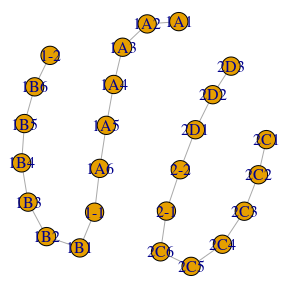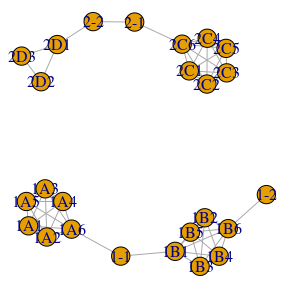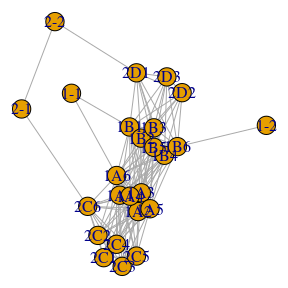# Running SConES

#### 2020-04-27

In this example we will analyze a simple case of GWAS data. We start by loading the martini, which contains the example we will work on.

library(martini)

# Experimental data

minigwas is a simplification of an actual GWAS. It is a list equivalent to what snpStats::read.pedfile would generate. In this experiment, we sequenced 25 SNPs on 100 samples. Half of them suffer from a disease, the other half are perfectly healthy individuals. The information of each patient is available in the fam element:

head(minigwas$fam) ## pedigree member father mother sex affected ## 1 1 1 NA NA 1 2 ## 2 2 2 NA NA 2 2 ## 3 3 3 NA NA 1 2 ## 4 4 4 NA NA 1 2 ## 5 5 5 NA NA 1 2 ## 6 6 6 NA NA 2 2 We pay close attention to the affected column, with takes value 2 if the patient is a case, or 1 if they are control. The other important element is map, which contains the SNP information: head(minigwas$map)
##   chr snp.names cm gpos allele.1 allele.2
## 1   1       1A1  0   10        A        G
## 2   1       1A2  0   20        A        G
## 3   1       1A3  0   30        A        G
## 4   1       1A4  0   40        A        G
## 5   1       1A5  0   50        A        G
## 6   1       1A6  0   60        A        G

In minigwas, the SNP name is a three character word, where every letter has a meaning: the first character indicates the chromosome, the second the gene, and the third its position inside the gene.

Lastly, genotypes contains the actual genotype for each SNP and sample.

minigwas\$genotypes
## A SnpMatrix with  100 rows and  25 columns
## Row names:  1 ... 100
## Col names:  1A1 ... 2D3

# The network

Other than the information from the GWAS experiment, we need a network of SNPs to work on. We can create those described in (Azencott et al. 2013) with the family of get_G*_network functions. Let’s see how they work.

gs <- get_GS_network(minigwas)
par(mar=c(0,0,0,0)+.1)
plot(gs)The GS network contains information about the genomic structure: it is created by connecting every SNP to the adjacent one. The result are one linear subgraph for each chromosome. Hence, GS network contains spacial information about the SNPs, which might be useful in investigating genotypes were a chromosomic region has been related to a disease.

gm <- get_GM_network(minigwas, snpMapping = minisnpMapping)
par(mar=c(0,0,0,0)+.1)
plot(gm)The GM network builds on the GS network, additionally interconnecting all SNPs belonging to the same gene. Still, each chromosome has its independent subnetwork. GM network gives insights into the functionality implications, as it will interconnect causal SNPs that belong to the same gene (which were already close in the GS network).

gi <- get_GI_network(minigwas, snpMapping = minisnpMapping, ppi = minippi)
par(mar=c(0,0,0,0)+.1)
plot(gi)The GI network builds on the GM network by connecting SNPs belonging to genes interacting with each other, for example, through a protein-protein interaction of their products. This kind of network contains, but it is not limited to, pathway information and crosstalk.

# Running SConES

Once we have the network, we can find ConES (connected explanatory SNPs), the set of SNPs $$S$$ that solve the problem

$argmax_{S \subseteq V} \sum_{p \in S} c_p - \sum_{p \in S} \eta - \sum_{p \in S} \sum_{q \notin S} \lambda W_{pq} .$ where $$c$$ represents an association measure with the phenotype (e.g. $$\chi^2_1$$) and $$W_{pq}$$ is the weight of the edge between $$p$$ and $$q$$. $$\eta$$ and $$\lambda$$ are parameters that enforce sparsity and connectivity on the subset respectively. Essentially, we are looking for a small subset of SNPs maximally associated with the phenotype that is mostly interconnected among itself in the network.

We can find ConES in martini with the function scones, which implements SConES (Azencott et al. 2013). By default, it takes a GWAS experiment and a SNP network, uses $$\chi^2_1$$ as association measure and performs a grid search over $$\eta$$ and $$\lambda$$ to find the combination that results in the best BIC.

cones <- search_cones(minigwas, gi)
## [0;32mComputing univariate association.[0m
## [0;34mSelecting the best hyperparameters...[0m
## [0;32mRunning models.[0m
## [0;32mFinding best model.[0m
## [0;34mSearching ConES with eta = 1e-06 and lambda = 1e-07
## [0m
## eta = 1e-06
## lambda = 1e-07

scones informs us of the optimal values for $$\eta$$ and $$\lambda$$. The results is a data frame that looks like this:

head(cones)
##   snp chr cm pos allele.1 allele.2        c selected module
## 3 1A1   1  0  10        A        G 95.48869     TRUE      1
## 4 1A2   1  0  20        A        G 95.48869     TRUE      1
## 5 1A3   1  0  30        A        G 94.15686     TRUE      1
## 6 1A4   1  0  40        A        G 96.15385     TRUE      1
## 7 1A5   1  0  50        A        G 94.15686     TRUE      1
## 8 1A6   1  0  60        A        G 93.35843     TRUE      1

The first six columns of the data frame come from the map data frame. The remaining represent the output of SConES:

• c contains the SNP univariate association score.
• selected is a logical vector indicating wether a SNP has been selected or not.
• module is an id shared by all the SNPs included in the same submodule of the solution subnetwork. They are not necesarily connected to each other, but there must be a path connecting them inside the solution subnetwork.